# RS Aggarwal Solutions for Class 6 Maths Chapter 2 Factors and Multiples Exercise 2C

RS Aggarwal Solutions for Class 6 Maths, Chapter 2, Factors and Multiples Exercise 2C helps students to understand the concepts like prime factorization and the concept of power to perform well in the class 6 exam. Students are advised to practice exercise wise problems using the solutions created by subject matter experts at BYJU’S.

Students who practice exercise wise problems using RS Aggarwal Solutions score high marks in examinations and also improve their knowledge in Mathematics. It mainly helps students to expertise in the subject and have a better understanding of the concepts.

## Download PDF of RS Aggarwal Solutions for Class 6 Maths Chapter 2 Factors and Multiples Exercise 2C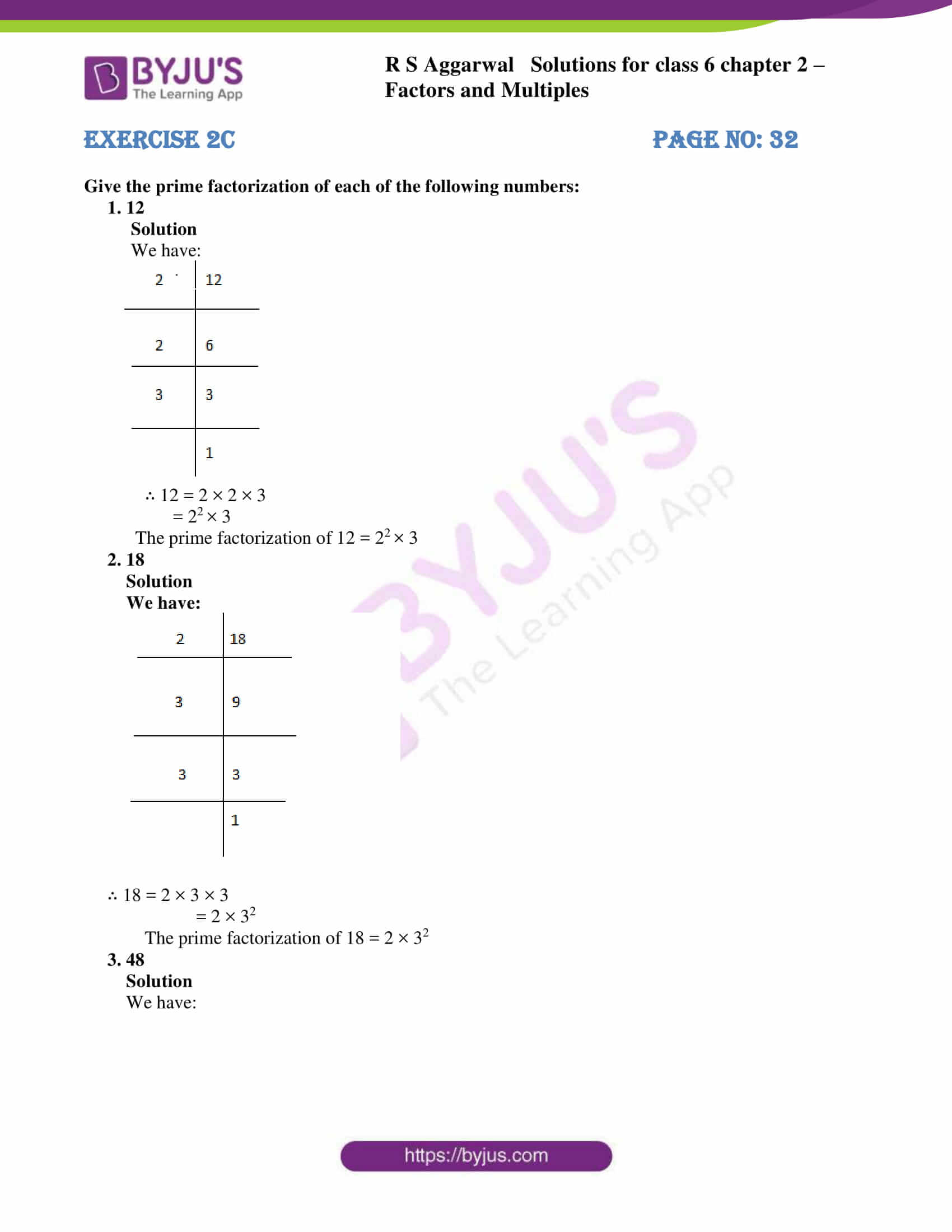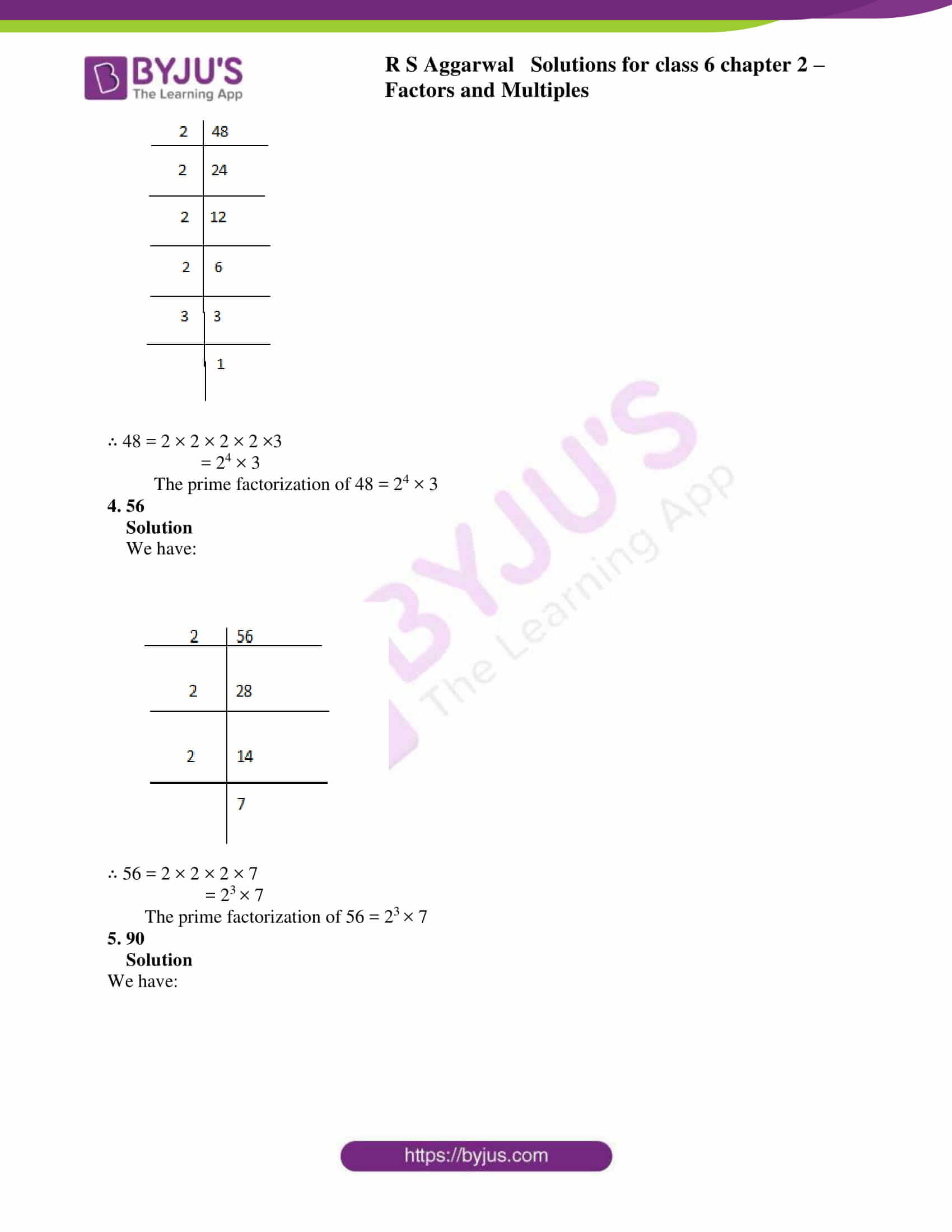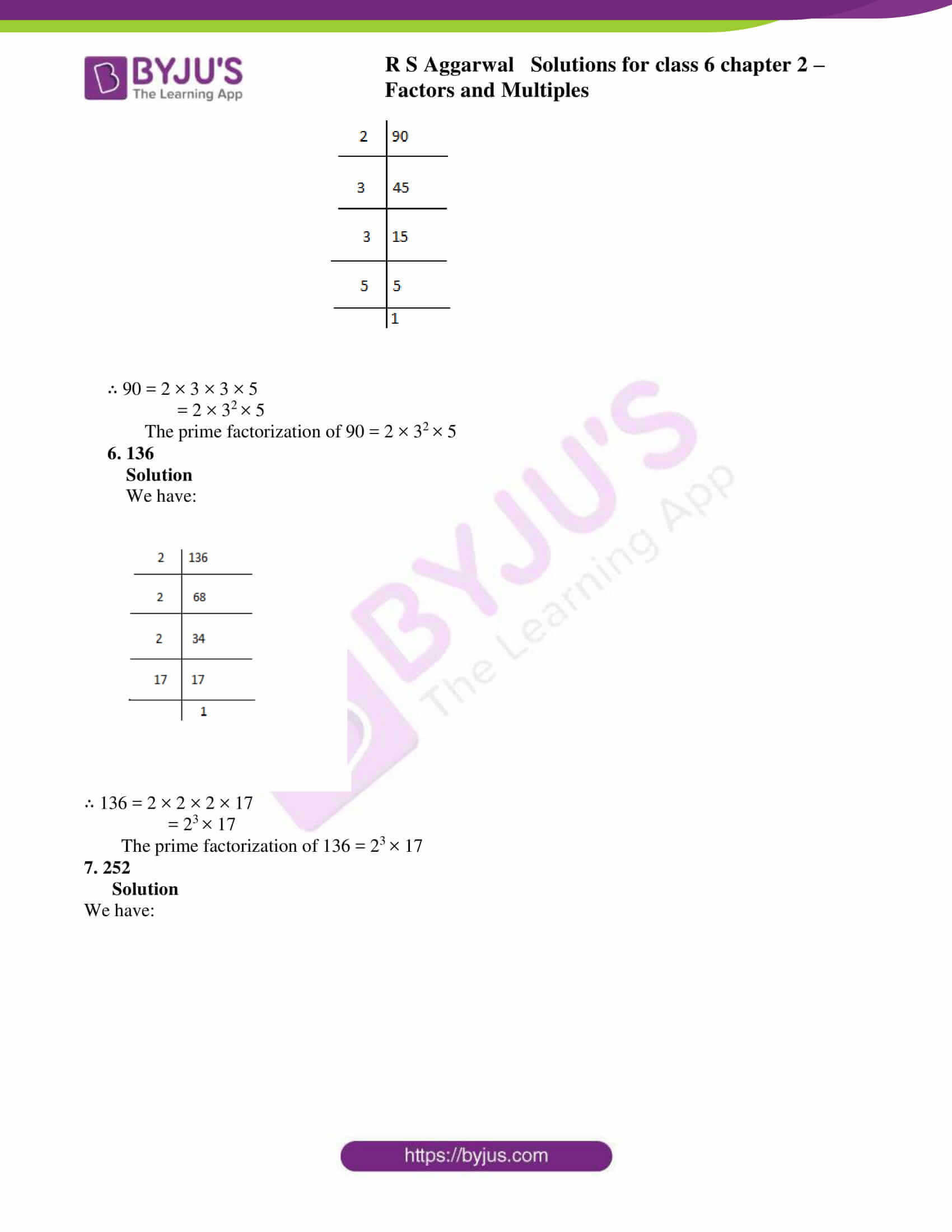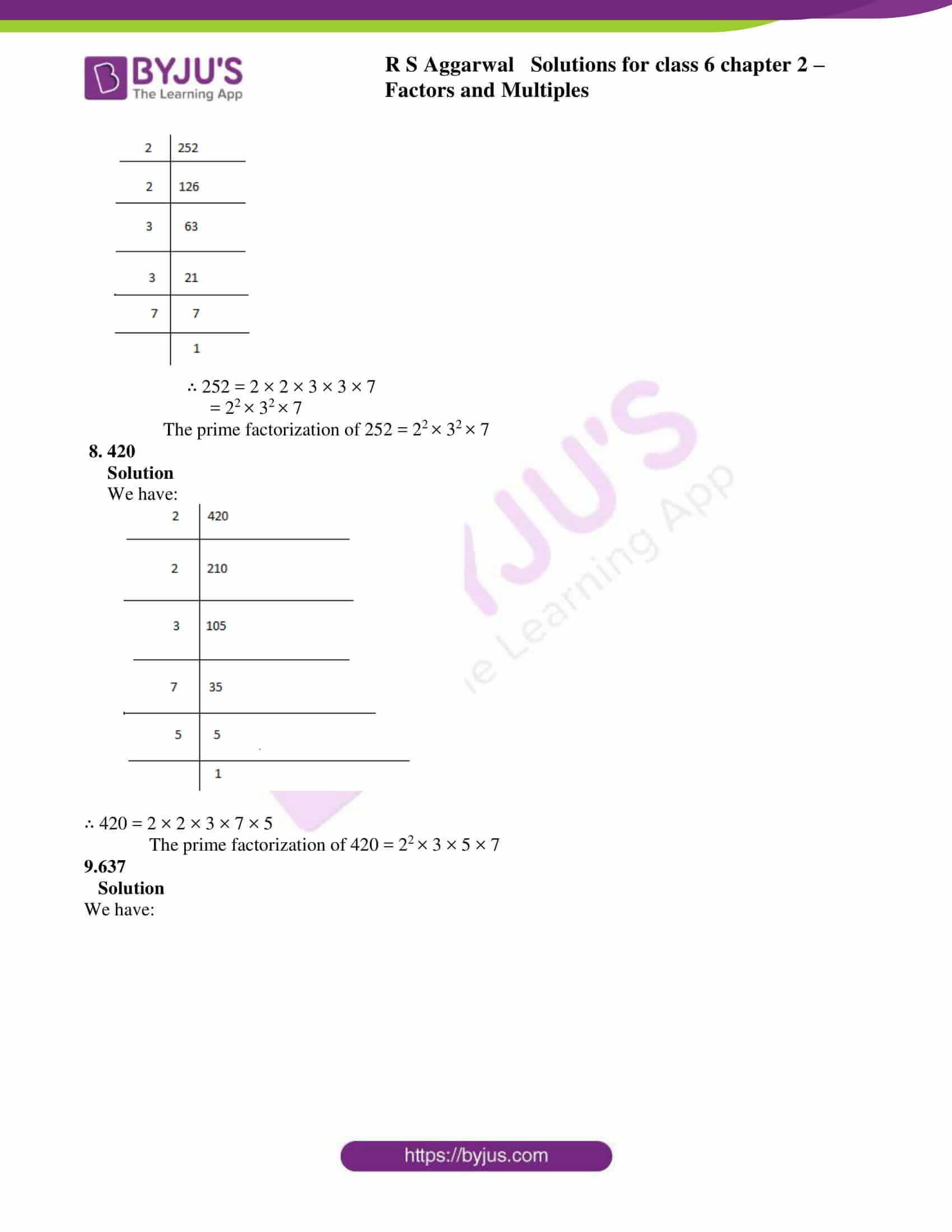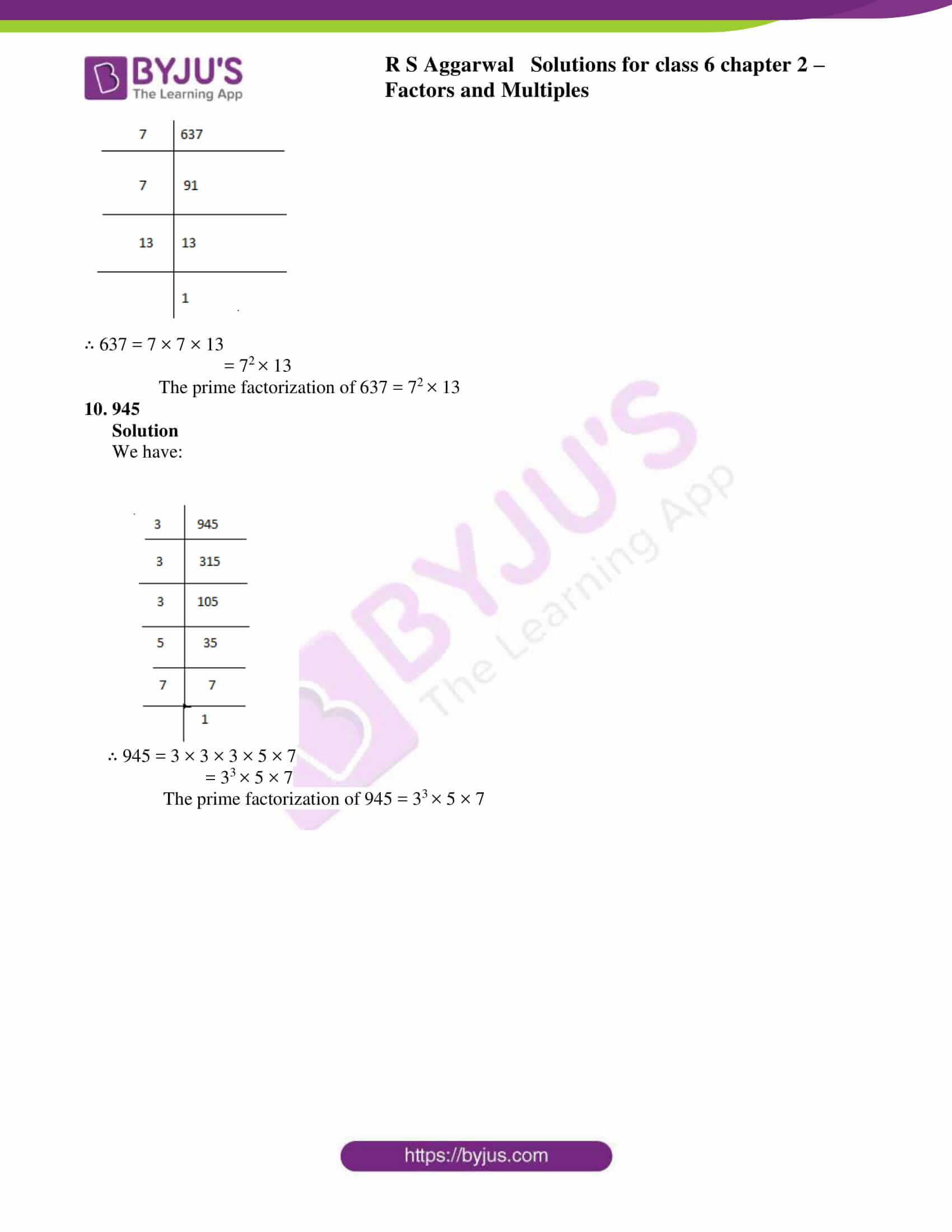### Access answers to Maths RS Aggarwal Solutions for Class 6 Chapter 2 Factors and Multiples Exercise 2C

Give the prime factorization of each of the following numbers:

1. 12

Solution

We have: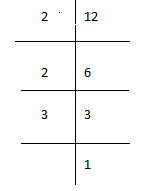∴ 12 = 2 × 2 × 3

= 22 × 3

The prime factorization of 12 = 22 × 3

2. 18

Solution

We have: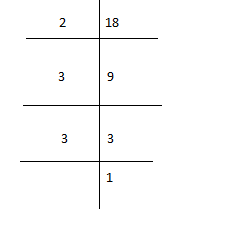∴ 18 = 2 × 3 × 3

= 2 × 32

The prime factorization of 18 = 2 × 32

3. 48

Solution

We have: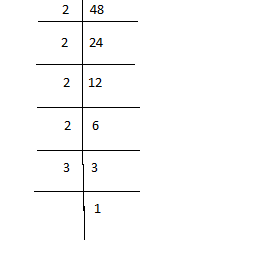∴ 48 = 2 × 2 × 2 × 2 ×3

= 24 × 3

The prime factorization of 48 = 24 × 3

4. 56

Solution

We have: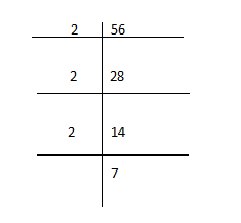∴ 56 = 2 × 2 × 2 × 7

= 23 × 7

The prime factorization of 56 = 23 × 7

5. 90

Solution

We have: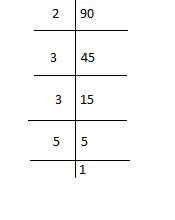∴ 90 = 2 × 3 × 3 × 5

= 2 × 32 × 5

The prime factorization of 90 = 2 × 32 × 5

6. 136

Solution

We have: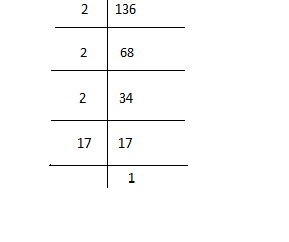∴ 136 = 2 × 2 × 2 × 17

= 23 × 17

The prime factorization of 136 = 23 × 17

7. 252

Solution

We have: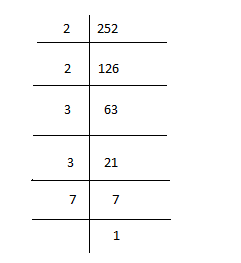∴ 252 = 2 × 2 × 3 × 3 × 7

= 22 × 32 × 7

The prime factorization of 252 = 22 × 32 × 7

8. 420

Solution

We have: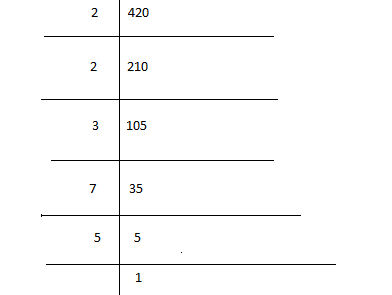∴ 420 = 2 × 2 × 3 × 7 × 5

The prime factorization of 420 = 22 × 3 × 5 × 7

9.637

Solution

We have: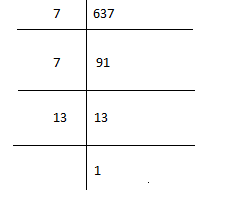∴ 637 = 7 × 7 × 13

= 72 × 13

The prime factorization of 637 = 72 × 13

10. 945

Solution

We have: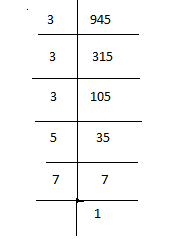∴ 945 = 3 × 3 × 3 × 5 × 7

= 33 × 5 × 7

The prime factorization of 945 = 33 × 5 × 7

## RS Aggarwal Solutions for Class 6 Maths Chapter 2 Factors and Multiples Exercise 2C

RS Aggarwal Solutions for Class 6 Maths Chapter 2 Factors and Multiples Exercise 2C has questions which are based on the topic prime factorization and concept of power. The students can make use of solutions PDF to understand prime factorization in depth. Other topics prior to this exercise are

• Finding prime and composite numbers
• General properties of divisibility
• To find HCF
• Properties of HCF and LCM of given numbers

Finishing homework has also become easy for the students by referring RS Aggarwal Solutions for Class 6. This helps them to strengthen their confidence in Mathematics and score high marks in the examinations.